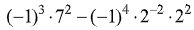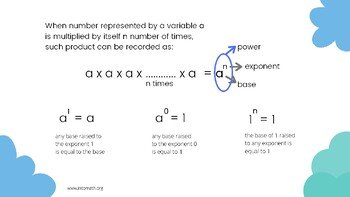CA$2.00 Exponents are very important and powerful. Read this blog post based on an ancient Indian legend about the creator of chess. This lesson teaches about exponents. An exponent shows how many times the number needs to be multiplied by itself. For example, 5³ = 5 × 5 × 5 = 125. In the order of operations, BEDMAS, exponents come right after operations in brackets. Sometimes exponents are used with variables. Then, exponent laws are used in order to simplify expressions with variables. This lesson explains how to use general exponent laws and provides insight into operations with exponents.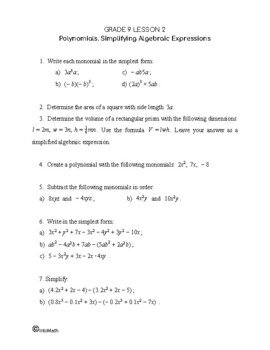Grade 9 Workbook (14 pages with answers) CA$4.00

Negative exponents make the base turn into its reciprocal.

Any number (except 0) or an algebraic term raised to the exponent of 0 is equal to 1.

When raising a fraction to an exponent it is necessary to raise both the numerator and the denominator to that exponent separately.

### Exponents and exponent laws short animation

Evaluate the following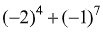Evaluate the following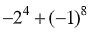Evaluate the following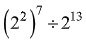Evaluate the following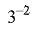Evaluate the following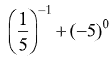Evaluate the following